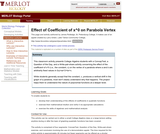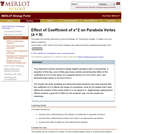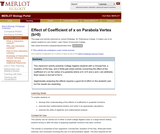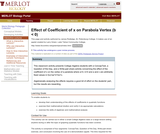# نتائج البحث (6)

View
Selected filters:
• Starting Point (SERC)Conditions of Use:
Remix and Share
Rating

The Daisy World model is intended to illustrate a mechanism through which biota might optimize their environment by means of negative feedback. The model offers a very simplified approach to a feedback system and can provide an introductory lesson in how models work. The aim of the model is to implement and test a mathematical model describing possible influence of biota on an abiotic (climatic) system using GAWK and GNUPLOT. The model tests the hypothesis that biota can influence the planetary environment. This site contains the online model of Daisy World for use in introductory courses as well as an assignment based on the model.

Material Type:
Interactive
Teaching/Learning Strategy
Provider:
Science Education Resource Center (SERC) at Carleton College
Provider Set:
Starting Point (SERC)
Author:
Robert Mackay
10/23/2006Conditions of Use:
يمكن إعادة ترتيب المحتوى ومشاركته
Rating

This classroom activity presents College Algebra students with a ConcepTest, a Question of the Day, and a Write-pair-share activity concerning the effect of the coefficient of x^0 (i.e., the constant, c) on the vertex of a parabola where a and b are arbitrarily fixed values in f(x)=ax^2+bx+c.

نوع المادة:
Activity/Lab
التقييم
Provider:
Science Education Resource Center (SERC) at Carleton College
Provider Set:
Pedagogy in Action
نقطة البداية (مركز الموارد لتعليم العلوم SERC)
المؤلف:
James J. Rutledge
08/28/2012Conditions of Use:
يمكن إعادة ترتيب المحتوى ومشاركته
Rating

This classroom activity presents College Algebra students with a ConcepTest, a Question of the Day, and a Write-pair-share activity concerning the effect of the coefficient of x^2 on the vertex of a parabola where a>0, b>0 and b and c are fixed values in f(x)=ax^2+bx+c.

نوع المادة:
Activity/Lab
التقييم
Provider:
Science Education Resource Center (SERC) at Carleton College
Provider Set:
Pedagogy in Action
نقطة البداية (مركز الموارد لتعليم العلوم SERC)
المؤلف:
James J. Rutledge
08/28/2012Conditions of Use:
يمكن إعادة ترتيب المحتوى ومشاركته
Rating

This classroom activity presents College Algebra students with a ConcepTest, a Question of the Day, and a Write-pair-share activity concerning the effect of the coefficient of x^2 on the vertex of a parabola where a<0, b>0 and b and c are fixed values in f(x)=ax^2+bx+c.

نوع المادة:
Activity/Lab
التقييم
Provider:
Science Education Resource Center (SERC) at Carleton College
Provider Set:
Pedagogy in Action
نقطة البداية (مركز الموارد لتعليم العلوم SERC)
المؤلف:
James J. Rutledge
08/28/2012Conditions of Use:
يمكن إعادة ترتيب المحتوى ومشاركته
Rating

This classroom activity presents College Algebra students with a ConcepTest, a Question of the Day, and a Write-pair-share activity concerning the effect of the coefficient of x on the vertex of a parabola where a>0, b>0 and a and c are fixed values in f(x)=ax^2+bx+c.

نوع المادة:
Activity/Lab
التقييم
Provider:
Science Education Resource Center (SERC) at Carleton College
Provider Set:
Pedagogy in Action
نقطة البداية (مركز الموارد لتعليم العلوم SERC)
المؤلف:
James J. Rutledge
08/28/2012Conditions of Use:
يمكن إعادة ترتيب المحتوى ومشاركته
Rating

This classroom activity presents College Algebra students with a ConcepTest, a Question of the Day, and a Write-pair-share activity concerning the effect of the coefficient of x on the vertex of a parabola where a>0, b<0 and a and c are fixed values in f(x)=ax^2+bx+c.

نوع المادة:
Activity/Lab
التقييم
Provider:
Science Education Resource Center (SERC) at Carleton College
Provider Set:
Pedagogy in Action
نقطة البداية (مركز الموارد لتعليم العلوم SERC)
المؤلف:
James J. Rutledge